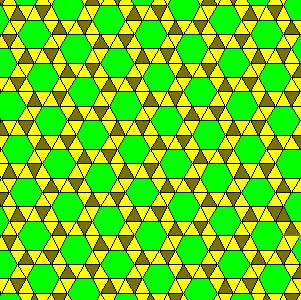# Snub trihexagonal tiling

Updated on
Covid-19
 Faces  irregular pentagonsRotation group  p6, [6,3], (632) Symmetry group  p6, [6,3], (632)Face configuration  V3.3.3.3.6Type  Dual semiregular tilingDual polyhedron  Snub trihexagonal tiling

In geometry, the snub hexagonal tiling (or snub trihexagonal tiling) is a semiregular tiling of the Euclidean plane. There are four triangles and one hexagon on each vertex. It has Schläfli symbol of sr{3,6}. The snub tetrahexagonal tiling is a related hyperbolic tiling with Schläfli symbol sr{4,6}.

## Contents

Conway calls it a snub hextille, constructed as a snub operation applied to a hexagonal tiling (hextille).

There are 3 regular and 8 semiregular tilings in the plane. This is the only one which does not have a reflection as a symmetry.

There is only one uniform coloring of a snub trihexagonal tiling. (Naming the colors by indices (3.3.3.3.6): 11213.)

## Circle packing

The snub trihexagonal tiling can be used as a circle packing, placing equal diameter circles at the center of every point. Every circle is in contact with 5 other circles in the packing (kissing number). The lattice domain (red rhombus) repeats 6 distinct circles. The hexagonal gaps can be filled by exactly one circle, leading to the densest packing from the triangular tiling#circle packing.

## Symmetry mutations

This semiregular tiling is a member of a sequence of snubbed polyhedra and tilings with vertex figure (3.3.3.3.n) and Coxeter–Dynkin diagram . These figures and their duals have (n32) rotational symmetry, being in the Euclidean plane for n=6, and hyperbolic plane for any higher n. The series can be considered to begin with n=2, with one set of faces degenerated into digons.

## Floret pentagonal tiling

In geometry, the floret pentagonal tiling or rosette pentagonal tiling is a dual semiregular tiling of the Euclidean plane. It is one of 15 known isohedral pentagon tilings. It is given its name because its six pentagonal tiles radiate out from a central point, like petals on a flower. Conway calls it a 6-fold pentille. Each of its pentagonal faces has four 120° and one 60° angle.

It is the dual of the uniform tiling, snub trihexagonal tiling, and has rotational symmetry of orders 6-3-2 symmetry.

## Variations

The floret pentagonal tiling has geometric variations with unequal edge lengths and rotational symmetry, which is given as monohedral pentagonal tiling type 5. In one limit, an edge-length goes to zero and it becomes a deltoidal trihexagonal tiling.

Similar Topics
Skyscraper Caper
Tim Atchison
David Mitogo
Topics